# Slender Beam with Variable Cross SectionThis test is used to validate the following attributes:

• Variable cross section

• Plane bending

• Natural frequencies.

You will use a 1D mesh.Reference:

Guide de validation des prologiciels de calcul des structrues, AFNOR technique, pp 200-201, 1990, SFM.

##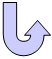Specifications

### Geometry Specifications

 Length: L = 1000 mm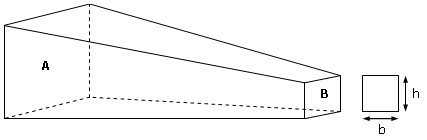Height and Width: h a = b a 40 mm h b = b b 10 mm

### Analysis Specifications

 Young Modulus (material): E = 200 GPa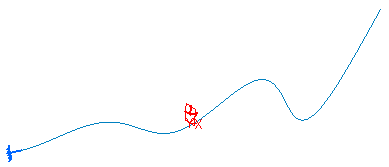Poisson's Ratio (material): ν = 0.3 Density: ρ = 7800 kg/m3 Mesh Specifications: 64 elements Restraints: Clamp on A: Tx = Ty = Tz = Rx = Ry = Rz = 0

##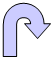Results

The beam bending differential equation (Euler-Bernoulli theory):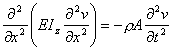The frequencies are given by the following formula: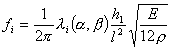where: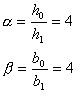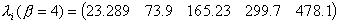So the exact frequencies are: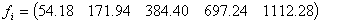The computed results for different numbers of elements are presented in the table below.

 Mode Analytical solution [Hz] 8 elements 16 elements 32 elements 64 elements 1 and 2 54.18 52.94 53.83 54.05 54.10 3 and 4 171.94 165.44 169.81 170.93 171.21 5 and 6 384.40 366.74 377.55 380.39 381.11 7 and 8 697.24 661.30 680.41 685.59 686.94 9 and 10 1112.28 1048.83 1077.38 1085.04 1087.13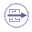To Perform the Test:

The SlenderBeamVariable.CATAnalysis document presents a complete analysis of this case, computed with a mesh formed of beam elements. Proceed as follow:

1. Open the CATAnalysis document.

2. Enter the Advanced Meshing Tools workbench.

3. In the specification tree, double-click the mesh part. The Global Parameters dialog box appears.

4. Change the mesh specifications as indicated in the Analysis Specifications table.

5. Compute the case in the Generative Structural Analysis workbench.